The perfect place for easy learning
Email address *
Question 1: What is the output of the following code?
1 point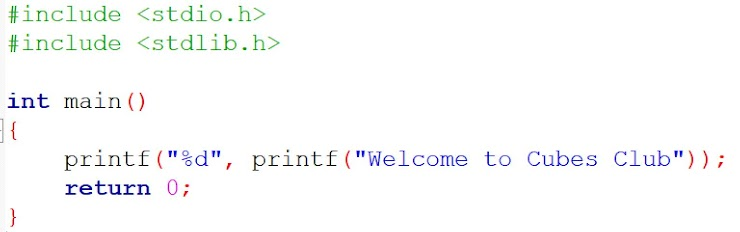Question 2: What is the output of the following code for the values of a and b are 10 & 20?
1 point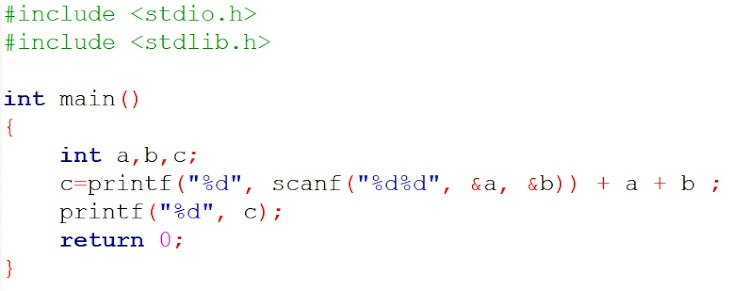Question 3: The format identifier ‘%i’ is also used for _____ data type.
1 point
Question 4: What is the output of the following code?
1 point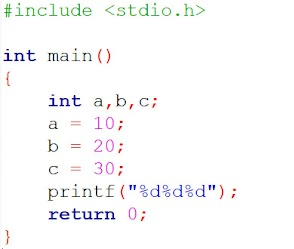Question 5: What is the output of the following code?
1 point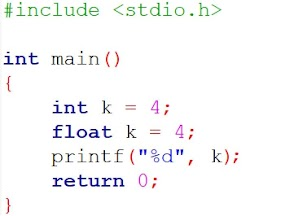Question 6: the 'break' can't be used with the following statements?
1 point
Question 7: What is the output of the following code?
1 point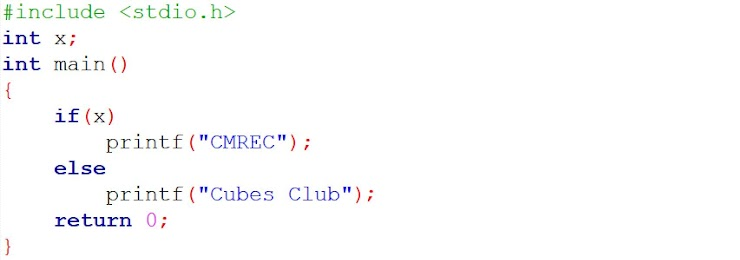Question 8: What is the output of the following code?
1 point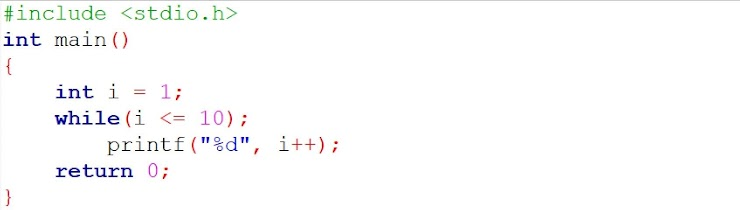Question 9: What is the output of the following code?
1 point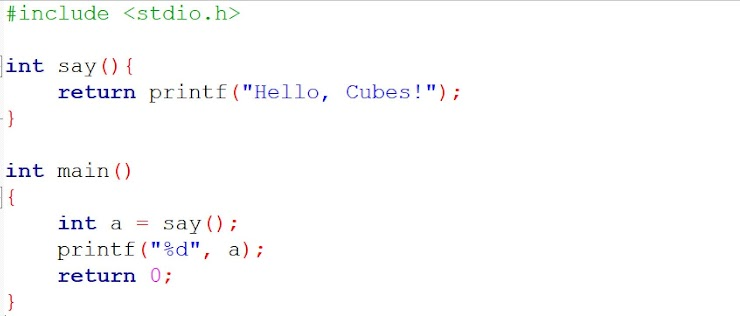Question 10: What is the output of the following code?
1 point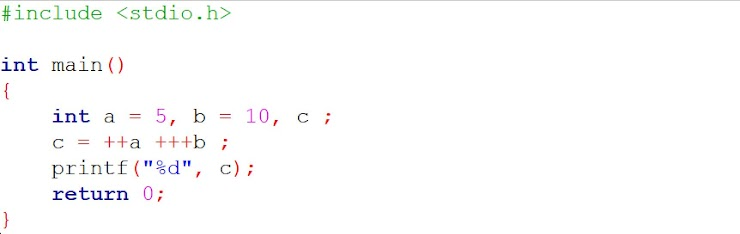Question 11: What is the output of the following code?
1 point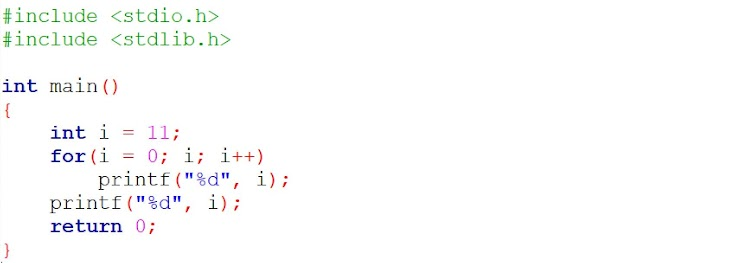Question 12: What is the output of the following code?
1 point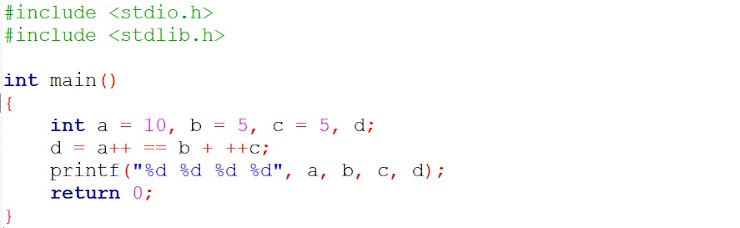Question 13: What is the output of the following code?
1 point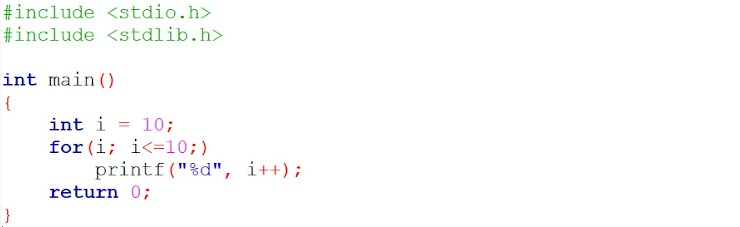Question 14: Pointer is used to store _____________.
1 point
Question 15: What is the output of the following code?
1 pointEnter Your Name *
Your answer
Enter Your Mobile Number *
Your answer
A copy of your responses will be emailed to the address you provided.
Never submit passwords through Google Forms.
reCAPTCHA
This content is neither created nor endorsed by Google. Report Abuse - Terms of Service